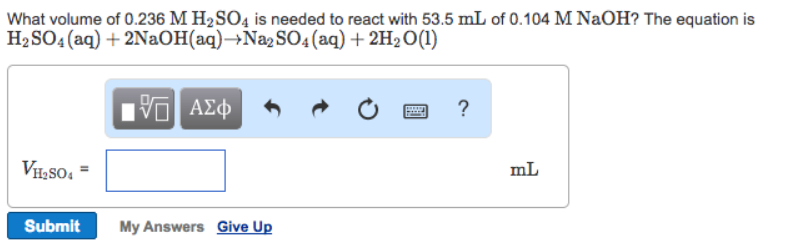# Problem: What volume of 0.236 M H2SO4 is needed to react with 53.5 mL of 0.104 M NaOH? The equation is H2SO4 aq) + 2NaOH (aq) → Na2SO4 (aq) + 2H2O (l)

###### FREE Expert Solution
86% (386 ratings)###### Problem Details

What volume of 0.236 M H2SO4 is needed to react with 53.5 mL of 0.104 M NaOH? The equation is

H2SO4 aq) + 2NaOH (aq) → Na2SO4 (aq) + 2H2O (l)